## Droz-Farny CirclesDraw a Circle with centerwhich cuts the lines,, and(where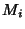are the Midpoints) at,;,; and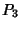,respectively, thenConversely, if equal Circles are drawn about the Vertices of a Triangle, they cut the lines joining the Midpoints of the corresponding sides in six points. These points lie on a Circle whose center is the Orthocenter. Ifis the Radius of the equal Circles centered on the vertices,, and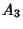, andis the Radius of the Circle about, then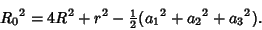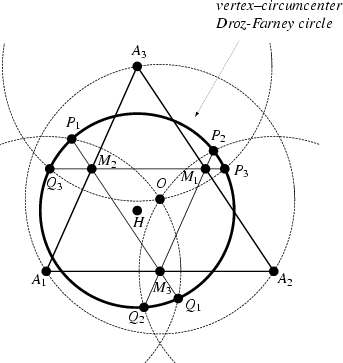If the circles equal to the Circumcircle are drawn about the Vertices of a triangle, they cut the lines joining midpoints of the adjacent sides in points of a Circlewith centerand Radius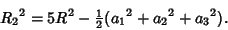It is equivalent to the circle obtained by drawing circles with centers at the feet of the altitudes and passing through the Circumcenter. These circles cut the corresponding sides in six points on a circlewhose center is.Furthermore, the circles about the midpoints of the sides and passing thoughcut the sides in six points lying on another equivalent circlewhose center is. In summary, the second Droz-Farny circle passes through 12 notable points, two on each of the sides and two on each of the lines joining midpoints of the sides.

References

Goormaghtigh, R. Droz-Farny's Theorem.'' Scripta Math. 16, 268-271, 1950.

Johnson, R. A. Modern Geometry: An Elementary Treatise on the Geometry of the Triangle and the Circle. Boston, MA: Houghton Mifflin, pp. 256-258, 1929.